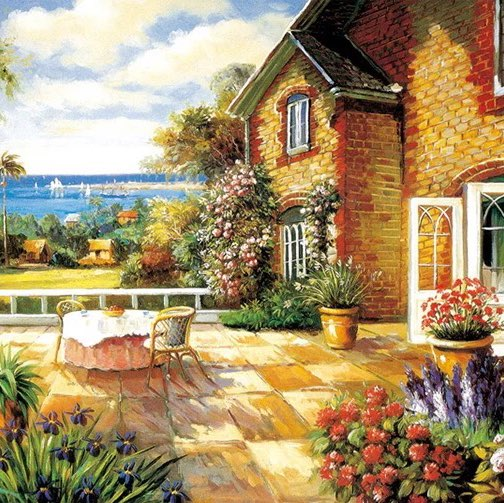Hi,Joe
SSAT数学考试真题分享Alexa 2016-11-04 16:38:10 发表于  [  资料共享  ]
587     0

1. What will it cost to carpet a room with indoor/outdoor carpet if the room is 10 feet wide and 12 feet long? The carpet costs 12.51 per square yard.

A. \$166.80

B. \$175.90

C. \$184.30

D. \$189.90

E. \$192.20

2. If the perimeter of a rectangular house is 44 yards, and the length is 36 feet, what is the width of the house?

A. 10 yards

B. 18 yards

C. 28 feet

D. 32 feet

E. 36 yards

3. What is the volume of the following cylinder?

A. 210.91

B. 226.20

C. 75.36

D. 904.32

E. 28.26

4. What is the volume of a cube whose width is 5 inches?

A. 15 cubic inches

B. 25 cubic inches

C. 64 cubic inches

D. 100 cubic inches

E. 125 cubic inches

5. Sally has three pieces of material. The first piece is 1 yd. 2 ft. 6 in. long, the second piece is 2 yd. 1 ft. 5 in long, and the third piece is 4 yd. 2ft.8in long. How much material does Sally have?

A. 7 yd. 1 ft. 8 in.

B. 8 yd. 4 ft. 4 in.

C. 8 yd. 11 in.

D. 9 yd. 7 in.

E. 10 yd.

6. A can´s diameter is 3 inches, and its height is 8 inches. What is the volume of the can?

A. 50.30

B. 56.55

C. 75.68

D. 113.04

E. 226.08Alexa 2021-06-15 09:28:52 重新编辑

### 评 论（ 0 ）### 我的评论